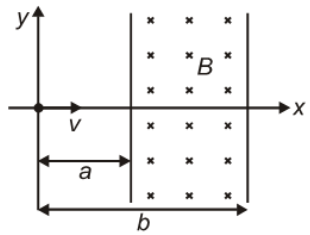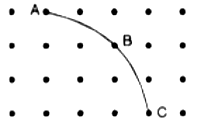The figure shows a particle of charge q and mass m moving with velocity v along the x-axis enters a region of the uniform magnetic field. The minimum value of v so that the charge q is deflected by an angle $30°$ is1.

2.

3.

4.  $\frac{\mathrm{qBb}}{2\mathrm{m}}$

Subtopic:  Lorentz Force |
To view explanation, please enrol in the course below.
NEET 2021 - Achiever Batch - Aryan Raj Singh

Difficulty Level:

An electron is traveling along the x-direction. It encounters a magnetic field in the y-direction. Its subsequent motion will be:

1.  Straight-line along the x-direction

2.  A circle in the xz-plane

3.  A circle in the yz-plane

4.  A circle in the xy-plane

Subtopic:  Lorentz Force |
To view explanation, please enrol in the course below.
NEET 2021 - Achiever Batch - Aryan Raj Singh

Difficulty Level:

A closed-loop (of any shape) carrying current is lying in the x-y plane. A uniform magnetic field B is present in the region such that the loop experiences zero force :

1.  B acts along the x-axis

2.  B acts along the y-axis

3.  B acts along the z-axis

4.  B can act along any of the above direction for the net force to be zero

Subtopic:  Current Carrying Loop: Force & Torque |
To view explanation, please enrol in the course below.
NEET 2021 - Achiever Batch - Aryan Raj Singh

Difficulty Level:

A galvanometer of resistance 240$\mathrm{\Omega }$ allows only 4% of the main current after connecting a shunt resistance. The value of shunt resistance is :

1.  10$\mathrm{\Omega }$

2.  20$\mathrm{\Omega }$

3.  8$\mathrm{\Omega }$

4.  5$\mathrm{\Omega }$

Subtopic:  Conversion to Ammeter & Voltmeter |
To view explanation, please enrol in the course below.
NEET 2021 - Achiever Batch - Aryan Raj Singh

Difficulty Level:

The dots in the figure show a magnetic field perpendicular to the plane of the paper and coming out of it. The curve ABC shows the trajectory of a particle in the plane of the paper. What is a particle?1.  Proton

2.  Electron

3.  Neutron

4.  It cannot be predicted

Subtopic:  Lorentz Force |
To view explanation, please enrol in the course below.
NEET 2021 - Achiever Batch - Aryan Raj Singh

Difficulty Level:

A long solenoid has 800 turns per meter length of the solenoid. A current of 1.6 A flows through it. The magnetic induction at the end of the solenoid on its axis is:

1.

2.

3.

4.

Subtopic:  Magnetic Field due to various cases |
To view explanation, please enrol in the course below.
NEET 2021 - Achiever Batch - Aryan Raj Singh

Difficulty Level:

The unit of reduction factor of the tangent galvanometer is

(1) Ampere

(2) Gauss

(4) None of these

Subtopic:  Moving Coil Galvanometer |
To view explanation, please enrol in the course below.
NEET 2021 - Achiever Batch - Aryan Raj Singh

Difficulty Level:

In order to pass 10% of the main current through a moving coil galvanometer of 99 ohms, the resistance of the required shunt is :

(1) 9.9 Ω

(2) 10 Ω

(3) 11 Ω

(4) 9 Ω

Subtopic:  Conversion to Ammeter & Voltmeter |
To view explanation, please enrol in the course below.
NEET 2021 - Achiever Batch - Aryan Raj Singh

Difficulty Level:

When a 12 Ω resistor is connected in parallel with a moving coil galvanometer then its deflection reduces from 50 divisions to 10 divisions. The resistance of the galvanometer is :

(1) 24 Ω

(2) 36 Ω

(3) 48 Ω

(4) 60 Ω

Subtopic:  Conversion to Ammeter & Voltmeter |
To view explanation, please enrol in the course below.
NEET 2021 - Achiever Batch - Aryan Raj Singh

Difficulty Level:

A voltmeter has a resistance of G ohms and range V volts. The value of resistance used in series to convert it into a voltmeter of range nV volts is :

(1) nG

(2) $\left(n-1\right)G$

(3) $\frac{G}{n}$

(4) $\frac{G}{\left(n-1\right)}$

Subtopic:  Conversion to Ammeter & Voltmeter |
To view explanation, please enrol in the course below.
NEET 2021 - Achiever Batch - Aryan Raj Singh

Difficulty Level: